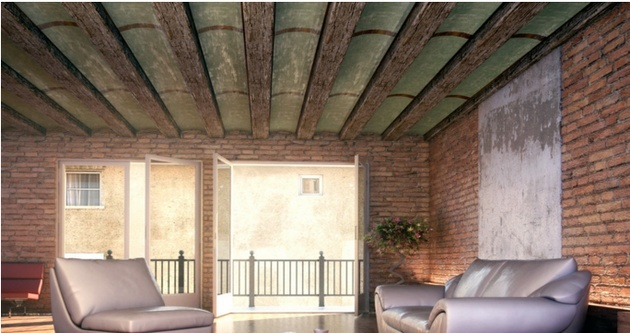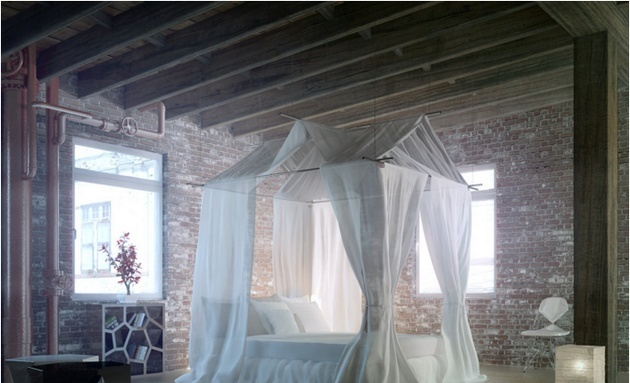|

# 精装房装修价格 精装房装修劣势

现如今，有很多业主选择精装修，在保证品质的同时，精装修的费用也是相对较高的，所以在选择精装修前一定要有基本预算，接下来，小编就给您介绍一下精装房装修价格 与精装房装修的劣势。精装房装修价格 精装房装修劣势

精装房装修价格

只要知道家庭精装修一平米多少钱，就可以计算出您的新房精装修的价格。?

1、墙基层处理每平米5元

2、墙体涂料粉刷墙衬加富亚六合一植物漆，每平米35元

3、地砖每平米大约为95元。因为会出现裁砖，破损等，所以地砖面积应该加3%--8%

砖挑选的简单方法：从包装箱中任意取出一片，看表面是否平整、完好，釉面、光亮、无斑点、缺釉、磕碰现象，四周边缘规整。釉面不光亮、发涩、或有气泡都属质量问题。再取出一片砖，两片对齐，中间缝隙越小越好。如果是图案砖必须用四片才能拼凑出一个完整图案来，还应看好砖的图案是否衔接、清晰。把这些砖一块挨一块竖起来，比较砖的尺寸是否一致，小砖偏差允许在正负1毫米，大砖允许在正负2毫米。

4、木地板使用仿实木每平米大约89元，如果要与地面找平，可以使用自流平水泥或者地宝，每平米大约加10元，总价每平米99元。地板报损加8%--10%。

5、厨房卫生间墙地砖每平米大约75元。报损与地面可以相同(墙面用普通工艺镶贴各种瓷片每平方米需普通水泥11kg、中砂33kg、石灰膏2kg。柱面上用普通工艺镶贴各种瓷片需普通水泥13kg、中砂27kg、石灰膏3kg。挑选方式可以参考地砖的方式。

6、卫生间设备每套大约4000元(坐便器、洗手盆、龙头、洗浴套件、镜子、纸盒、皂盒、毛巾杆、托盘、地漏、浴霸)。现在市场有一些比较便宜的卫具，但是质量相当的差，所以建议不要使用太便宜的。

7、厨房橱柜每延米大约1800元。(水晶板、亚克力、烤漆等，但是不包括品牌，还有龙头、水池)

8、吊顶每平米大约50元。

9、阳台墙地砖每平米80元，需要注意尽可能不要使用胶霸粘贴，因为味道过重。

10、阳台衣架巧太太每套230元，安装人员单收10元，总价为240元，记得索要保修凭据，因为这些东西是由厂家保修的。

11、套装门建议使用复合实木会结实一些，每套大约1250元，包括百乐门锁，加厚合页，门吸。如果不是因为资金问题建议不要使用贴面门的密度板门了，不太结实，如果一定要使用可以使用三套合页，也可以保持比较好的使用。

12、卫生间门建议使用高分子结构，可以很好的起到防水性，每套大约850元，配件与其它的相同。十三、卫生间防水每平米25元。

13、电力改造大约5500元。

14、灯具大约为3500元，一般中小型公司是不收安装费的，因为这部分钱已经在电力改造中。

精装房装修劣势

1、“精装修”缺乏明确和统一的标准

精装修中最大的弊病是精装修缺乏明确和统一的认定标准，这在相当程度上为一些不负责任的开发商利用“精装修”概念迷惑消费者提供了可能。之所以在各界呼声较高的情况下相关标准迟迟不能出台，主要原因在于精装修标准的制定是一个相当复杂的体系，由于各地经济水平、消费能力不均衡，不适合“一刀切”。

精装修与毛坯房交付的区别在于，毛坯房交房的装修标准是国家强制标准，如《住宅工程初装饰竣工验收办法》，而精装修房屋在交房时却没有国家强制执行的验收办法作为准绳，有的只是一些如《建筑装饰装修工程质量验收规范》等推荐标准。无论是“800元/平方米”还是“1000元/平方米”的装修标准，在没有统一验收规范的保证下都成了模糊概念。

在相关强制标准出台之前，消费者应该向开发商索要《商品房使用说明书》、《商品房质量保证书》，因为在这“二书”中对精装修产品的品牌规格、使用年限以及保修范围都进行了约定，消费者可以以此保障自身权益。

2、三大陷阱坑苦买房人

由于信息的不对称，消费者在选择精装修住宅时，容易对开发商的信誓旦旦听之信之，从而使自身利益无法得到保障，精装修住宅存在货不对板、质量低下、价值虚报三大陷阱。

货不对板：一般情况，开发商会故意将样板间装修标准高出实际规格。由于精装修住宅并不容易看出内部品质，质量问题往往会在使用中体现出来，成为精装修中最“隐蔽”的陷阱。

质量低下：而一些开发商提供的知名品牌并不假，只是他们会“偷梁换柱”选用这一品牌中的低档过时产品，而不明就里的消费者却为此付出合格产品的价格。由于普通购房者是很难甄别精装修的品质的，开发商借此降低施工质量标准、减少施工成本投入。

价值虚报：许多精装修住宅往往看上去很美，其实远远低于实际价值，开发商会一味夸大装修标准，而实际费用却低得离谱。另外，由于房产开发周期较长，等一两年交房后，装修时所用的产品早已贬值，而且开发商大规模采购也会降低成本，而这一部分盈余依然要消费者埋单。`声明：本文由入驻焦点开放平台的作者撰写，除焦点官方账号外，观点仅代表作者本人，不代表焦点立场错误信息举报电话： 400-099-0099，邮箱：jubao@vip.sohu.com，或点此进行意见反馈，或点此进行举报投诉。`A B C D E F G H J K L M N P Q R S T W X Y Z
A - B - C - D - E
• A
• 鞍山
• 安庆
• 安阳
• 安顺
• 安康
• 澳门
• B
• 北京
• 保定
• 包头
• 巴彦淖尔
• 本溪
• 蚌埠
• 亳州
• 滨州
• 北海
• 百色
• 巴中
• 毕节
• 保山
• 宝鸡
• 白银
• 巴州
• C
• 承德
• 沧州
• 长治
• 赤峰
• 朝阳
• 长春
• 常州
• 滁州
• 池州
• 长沙
• 常德
• 郴州
• 潮州
• 崇左
• 重庆
• 成都
• 楚雄
• 昌都
• 慈溪
• 常熟
• D
• 大同
• 大连
• 丹东
• 大庆
• 东营
• 德州
• 东莞
• 德阳
• 达州
• 大理
• 德宏
• 定西
• 儋州
• 东平
• E
• 鄂尔多斯
• 鄂州
• 恩施
F - G - H - I - J
• F
• 抚顺
• 阜新
• 阜阳
• 福州
• 抚州
• 佛山
• 防城港
• G
• 赣州
• 广州
• 桂林
• 贵港
• 广元
• 广安
• 贵阳
• 固原
• H
• 邯郸
• 衡水
• 呼和浩特
• 呼伦贝尔
• 葫芦岛
• 哈尔滨
• 黑河
• 淮安
• 杭州
• 湖州
• 合肥
• 淮南
• 淮北
• 黄山
• 菏泽
• 鹤壁
• 黄石
• 黄冈
• 衡阳
• 怀化
• 惠州
• 河源
• 贺州
• 河池
• 海口
• 红河
• 汉中
• 海东
• I
• J
• 晋中
• 锦州
• 吉林
• 鸡西
• 佳木斯
• 嘉兴
• 金华
• 景德镇
• 九江
• 吉安
• 济南
• 济宁
• 焦作
• 荆门
• 荆州
• 江门
• 揭阳
• 金昌
• 酒泉
• 嘉峪关
K - L - M - N - P
• K
• 开封
• 昆明
• 昆山
• L
• 廊坊
• 临汾
• 辽阳
• 连云港
• 丽水
• 六安
• 龙岩
• 莱芜
• 临沂
• 聊城
• 洛阳
• 漯河
• 娄底
• 柳州
• 来宾
• 泸州
• 乐山
• 六盘水
• 丽江
• 临沧
• 拉萨
• 林芝
• 兰州
• 陇南
• M
• 牡丹江
• 马鞍山
• 茂名
• 梅州
• 绵阳
• 眉山
• N
• 南京
• 南通
• 宁波
• 南平
• 宁德
• 南昌
• 南阳
• 南宁
• 内江
• 南充
• P
• 盘锦
• 莆田
• 平顶山
• 濮阳
• 攀枝花
• 普洱
• 平凉
Q - R - S - T - W
• Q
• 秦皇岛
• 齐齐哈尔
• 衢州
• 泉州
• 青岛
• 清远
• 钦州
• 黔南
• 曲靖
• 庆阳
• R
• 日照
• 日喀则
• S
• 石家庄
• 沈阳
• 双鸭山
• 绥化
• 上海
• 苏州
• 宿迁
• 绍兴
• 宿州
• 三明
• 上饶
• 三门峡
• 商丘
• 十堰
• 随州
• 邵阳
• 韶关
• 深圳
• 汕头
• 汕尾
• 三亚
• 三沙
• 遂宁
• 山南
• 商洛
• 石嘴山
• T
• 天津
• 唐山
• 太原
• 通辽
• 铁岭
• 泰州
• 台州
• 铜陵
• 泰安
• 铜仁
• 铜川
• 天水
• 天门
• W
• 乌海
• 乌兰察布
• 无锡
• 温州
• 芜湖
• 潍坊
• 威海
• 武汉
• 梧州
• 渭南
• 武威
• 吴忠
• 乌鲁木齐
X - Y - Z
• X
• 邢台
• 徐州
• 宣城
• 厦门
• 新乡
• 许昌
• 信阳
• 襄阳
• 孝感
• 咸宁
• 湘潭
• 湘西
• 西双版纳
• 西安
• 咸阳
• 西宁
• 仙桃
• 西昌
• Y
• 运城
• 营口
• 盐城
• 扬州
• 鹰潭
• 宜春
• 烟台
• 宜昌
• 岳阳
• 益阳
• 永州
• 阳江
• 云浮
• 玉林
• 宜宾
• 雅安
• 玉溪
• 延安
• 榆林
• 银川
• Z
• 张家口
• 镇江
• 舟山
• 漳州
• 淄博
• 枣庄
• 郑州
• 周口
• 驻马店
• 株洲
• 张家界
• 珠海
• 湛江
• 肇庆
• 中山
• 自贡
• 资阳
• 遵义
• 昭通
• 张掖
• 中卫

1室1厅1厨1卫1阳台

1
2
3
4
5

0
1
2

1

1

0
1
2
3报名成功，资料已提交审核A B C D E F G H J K L M N P Q R S T W X Y Z
A - B - C - D - E
• A
• 鞍山
• 安庆
• 安阳
• 安顺
• 安康
• 澳门
• B
• 北京
• 保定
• 包头
• 巴彦淖尔
• 本溪
• 蚌埠
• 亳州
• 滨州
• 北海
• 百色
• 巴中
• 毕节
• 保山
• 宝鸡
• 白银
• 巴州
• C
• 承德
• 沧州
• 长治
• 赤峰
• 朝阳
• 长春
• 常州
• 滁州
• 池州
• 长沙
• 常德
• 郴州
• 潮州
• 崇左
• 重庆
• 成都
• 楚雄
• 昌都
• 慈溪
• 常熟
• D
• 大同
• 大连
• 丹东
• 大庆
• 东营
• 德州
• 东莞
• 德阳
• 达州
• 大理
• 德宏
• 定西
• 儋州
• 东平
• E
• 鄂尔多斯
• 鄂州
• 恩施
F - G - H - I - J
• F
• 抚顺
• 阜新
• 阜阳
• 福州
• 抚州
• 佛山
• 防城港
• G
• 赣州
• 广州
• 桂林
• 贵港
• 广元
• 广安
• 贵阳
• 固原
• H
• 邯郸
• 衡水
• 呼和浩特
• 呼伦贝尔
• 葫芦岛
• 哈尔滨
• 黑河
• 淮安
• 杭州
• 湖州
• 合肥
• 淮南
• 淮北
• 黄山
• 菏泽
• 鹤壁
• 黄石
• 黄冈
• 衡阳
• 怀化
• 惠州
• 河源
• 贺州
• 河池
• 海口
• 红河
• 汉中
• 海东
• I
• J
• 晋中
• 锦州
• 吉林
• 鸡西
• 佳木斯
• 嘉兴
• 金华
• 景德镇
• 九江
• 吉安
• 济南
• 济宁
• 焦作
• 荆门
• 荆州
• 江门
• 揭阳
• 金昌
• 酒泉
• 嘉峪关
K - L - M - N - P
• K
• 开封
• 昆明
• 昆山
• L
• 廊坊
• 临汾
• 辽阳
• 连云港
• 丽水
• 六安
• 龙岩
• 莱芜
• 临沂
• 聊城
• 洛阳
• 漯河
• 娄底
• 柳州
• 来宾
• 泸州
• 乐山
• 六盘水
• 丽江
• 临沧
• 拉萨
• 林芝
• 兰州
• 陇南
• M
• 牡丹江
• 马鞍山
• 茂名
• 梅州
• 绵阳
• 眉山
• N
• 南京
• 南通
• 宁波
• 南平
• 宁德
• 南昌
• 南阳
• 南宁
• 内江
• 南充
• P
• 盘锦
• 莆田
• 平顶山
• 濮阳
• 攀枝花
• 普洱
• 平凉
Q - R - S - T - W
• Q
• 秦皇岛
• 齐齐哈尔
• 衢州
• 泉州
• 青岛
• 清远
• 钦州
• 黔南
• 曲靖
• 庆阳
• R
• 日照
• 日喀则
• S
• 石家庄
• 沈阳
• 双鸭山
• 绥化
• 上海
• 苏州
• 宿迁
• 绍兴
• 宿州
• 三明
• 上饶
• 三门峡
• 商丘
• 十堰
• 随州
• 邵阳
• 韶关
• 深圳
• 汕头
• 汕尾
• 三亚
• 三沙
• 遂宁
• 山南
• 商洛
• 石嘴山
• T
• 天津
• 唐山
• 太原
• 通辽
• 铁岭
• 泰州
• 台州
• 铜陵
• 泰安
• 铜仁
• 铜川
• 天水
• 天门
• W
• 乌海
• 乌兰察布
• 无锡
• 温州
• 芜湖
• 潍坊
• 威海
• 武汉
• 梧州
• 渭南
• 武威
• 吴忠
• 乌鲁木齐
X - Y - Z
• X
• 邢台
• 徐州
• 宣城
• 厦门
• 新乡
• 许昌
• 信阳
• 襄阳
• 孝感
• 咸宁
• 湘潭
• 湘西
• 西双版纳
• 西安
• 咸阳
• 西宁
• 仙桃
• 西昌
• Y
• 运城
• 营口
• 盐城
• 扬州
• 鹰潭
• 宜春
• 烟台
• 宜昌
• 岳阳
• 益阳
• 永州
• 阳江
• 云浮
• 玉林
• 宜宾
• 雅安
• 玉溪
• 延安
• 榆林
• 银川
• Z
• 张家口
• 镇江
• 舟山
• 漳州
• 淄博
• 枣庄
• 郑州
• 周口
• 驻马店
• 株洲
• 张家界
• 珠海
• 湛江
• 肇庆
• 中山
• 自贡
• 资阳
• 遵义
• 昭通
• 张掖
• 中卫• 手机• 分享
• 设计
免费设计
• 计算器
装修计算器
• 入驻
合作入驻
• 联系
联系我们
• 置顶
返回顶部